Mathematical Means and Averages: Basic Properties by Stanislav Sýkora, Extra Byte, Via R.Sanzio 22C, Castano Primo, Italy 20022 in Stan's Library, Ed.S.Sykora, Vol.III. First release July 15, 2009 Permalink via DOI:  10.3247/SL3Math09.001
 Other math articles in Stan's Library | Math Links Math Books | Stan's HUB
 Abstract and Introduction This article can be used as a primer for students and non-mathematicians. I find the otherwise excellent introductions to means in Wikipedia and Math World not quite complete in some respects and not as easily accessible to a layman as they could/should be (in any case, the more such introductory sources there are, the better). Since my goal here is to present a bird-view perspective of the topic to a layman who probably wishes to skip the proofs, the latter are collected in an Appendix (please, read the two generic notes at the beginning of the Appendix before going through any of the proofs). The exposition stops short of discussing the applications of means in probability theory which is a huge topic to be handled in a separate treatise. Although the text is kept as simple as possible, it contains several original ideas which, I believe, enhance the internal coherence of the topic and, especially, its relevance to practical applications. The pertinent definitions are a little bit more rigorous than what one would expect in a primer and are formulated in a way which underlines the difference between means and averages. I have also tried and coined terms and definitions for several desirable properties of means and averages such as simplicity, regularity, stability, coherence, efficiency and thriftiness. Since these are de-facto simple concepts, I am sure that each of them has already been discussed somewhere either directly or indirectly, but, unfortunately, I have found it difficult to dug out the pertinent references and had to reinvent them from scratch. Next article in this series: Generalized Heronian means.

1. Intuitive definition of a mean

Let a ≡ {ak; k = 1, 2, ..., n} be a non-empty, finite subset of real numbers belonging to a domain D. The latter may be the set of all real numbers R, or the set of all positive real numbers R+, or that of non-negative real numbers R0+. Denoting as A the set of all such subsets, we call a mean any mapping MAD which has the following properties:

• (m1) Symmetry: M(P(a)) = M(a).
• (m2) Linear scaling: M(ca) = cM(a), where ca = {cak; k = 1, 2, ..., n} and c is any positive real number.
• (m3) Bounds: min(a) ≤ M(a) ≤ max(a).

Here min(a) and max(a) are respectively the minimum and the maximum values among the elements of the subset a,
and P(a) is any permutation of a.

The first condition says that a mean is invariant (symmetric) with respect to the permutations of the elements of a.
In other words, as far as means are concerned, the order of the elements of a is - and must be - completely irrelevant.

The second condition (a special case of functional homogeneity) is particularly significant in physical contexts where it guarantees a kind of linearity as well as the condition that a mean should have the same physical dimension as the elements ak. For example, any mean of weights is again a weight, and any mean of distances expressed in miles is again a distance expressed in miles.

The last condition implies, among other things, that when all the elements of a have the same value v, then any mean M(a) is also equal to v. In particular, for n = 1 we obtain that M(a) = a for any element a of D and any mean M. It also implies that if the elements of a all belong to a certain real numbers interval D, then so does the mean. As a consequence, a mean defined anywhere in R is also defined in any real numbers interval.

In practice, a particular mean in a domain D can be defined by a suitable expression which involves the values {ak} and their number n, and which is symmetric (invariant) with respect to any permutation of the indices k.

There are an infinity of means. For various reasons, most of which will be discussed later in this article, certain means have acquired a particular popularity and were studied in great detail.

2. Examples of means

This Section lists the most frequently used classical means. In all cases, it is quite simple to show that they indeed satisfy the defining conditions (m1,m2,m3) but, for coherence, formal proofs are anyway given in the Appendix. For the purposes of this Section the reader may skip them, but they are quite useful as college-level excercises.

Classical means on the domain of all real numbers R:

***** Minimum & Maximum:
Formally, min(a) ≡ min(a) and max(a) ≡ max(a), where the right-hand sides are the algebraic minimum and maximum functions, respectively. The mappings min and max both satisfy the defining conditions and therefore are means, even though they are rarely mentioned - or even perceived - as such.

***** Median::
The median med(a) of a is defined operationally as follows: First, sort (order) the elements of a so that, when k is the index of the k-th element after the sorting, ak ≤ ak+1 for any k = 1,2,...,n-1. Then
- When n is odd,  med(a) = a(n+1)/2
- When n is even, med(a) = (an/2+a1+n/2)/2

***** Arithmetic mean:
This is the best known and the most frequently used mean (to the point that it is sometimes improperly identified with the broader term mean). It is defined by the following algebraic expression:
(1)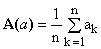***** Robust means are defined by recipes like the following one:
- Assume a value for a factor t (relative threshold) such that 0 ≤ t < 0.5 (typically, t = 0.05, or 5%).
- Eliminate [tn] largest elements of a and [tn] smallest ones (square brackets indicate the integer part of tn).
- Calculate the arithmetic average of the values which remain.

This case is an example of a mean which involves a real parameter (t) and thus represents a family of means.
Note: this definition of a robust mean is not unique; several slightly different ones are also in common use.

Classical means on the domains R+ and R0+:

***** Geometric mean, defined by

(2)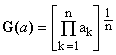.

***** Harmonic mean, defined by

(3)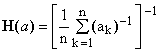.

When any of the elements of a is zero, the harmonic mean is defined to be also zero, in accordance with the corresponding limit.

***** Hölder means contain one non-zero real parameter p and are defined as

(4)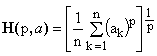For a few special values of p, Hölder mean coincides with special means which have names of their own:

min(a) = limp→-∞H(p,a).
H(a) = H(-1,a) is the harmonic mean .
G(a) = limp→0H(p,a) is the geometric mean.
A(a) = H(1,a) is the arithmetic mean of Eq.(1) .
Q(a) = H(2,a) is the quadratic mean .
max(a) = limp→+∞H(p,a).

Keeping the set a fixed and varying the value of p from minus infinity to plus infinity,
the Hölder mean H(p,a) traces what we will call the Hölder path of the set a.

Note: Hölder mean is sometimes called power mean or generalized mean. While the origin of both terms is comprehensible, they should be discouraged since they conflict with other types of means with an equal or superior right to such names (see the Section on generalized means below).

***** Lehmer means contain one non-zero real parameter p and are defined as

(5)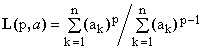For a few special values of p, Lehmer means coincide with these special means:

min(a) = limp→-∞L(p,a).
H(a) = L(0,a) is the harmonic mean .
A(a) = L(1,a) is the arithmetic mean of Eq.(1).
L(2,a) = Q2(a)/A(a)  (it is interesting to see that this ratio has all the properties of a mean).
max(a) = limp→+∞L(p,a).

Keeping the set a fixed and varying the value of p from minus infinity to plus infinity,
the Lehmer mean L(p,a) traces what we will call the Lehmer path of the set a.

Graphical examples of the classical means

Figure 1. Hölder mean H(p;a) and Lehmer mean L(p;a) as functions of p
for the set a = {1,2,3,4,5,6,7,8,9,10}.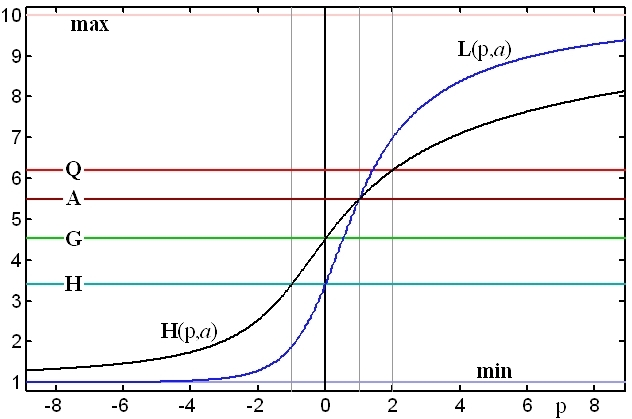The two lines trace the Hölder path (black) and the Lehmer path (blue). The horizontal lines indicate, for the same set a, the minimum (lowermost), the harmonic mean H, the geometric mean G, the arithmetic mean A, the quadratic mean Q and the maximum (uppermost).

3. Abstract definitions of means and averages

The terms mean value and average value are often used as synonyms but they are actually two quite different concepts.
The three examples which follow should help to clarify the difference.

Example 1: One can hardly object to the physical concept of an average force on a body, while there is something amiss in the hypothetical term mean force. The problem is that forces are 3D vectors and they can cancel even when non of them is zero. The idea of several non-zero entities averaging to zero is acceptable, but it conflicts with the intuitive notion of a mean value.

Example 2: A finite subset of complex numbers may have an average compatible with formula (1) but it is not a mean for, due to the lack of definition of a minimum (or a maximum) of a set of complex numbers, there is no way to check the condition (m3). Actually, this applies also to the preceding case and, vice versa, the objection of the previous example holds also for this one.

Example 3: Consider the quadratic mean of Eq.(4) with p=2, and apply it to a finite set of real numbers, admitting also negative values. The formula is then still legitimate and amounts to an average known as the root-mean-square. But it is not a mean since, for example, the rms of {1,-2} is positive and therefore, again, does not satisfy the condition (m3).

From these examples it is evident that the difference lies in the condition (m3) which needs to be satisfied by means but not by averages. We will now try and define the terms mean and average for as wide categories of sets as possible, exploiting the insight about (m3) for a formal distinction between the them.

Definition: Let e ≡ {ek; k = 1, 2, ..., n} be a non-empty, finite subset of a domain set S which satisfies some conditions to be defined later. Denoting as E the set of all such subsets, we call a mean any mapping MES satisfying the following conditions:

• M1) Symmetry: M(P(e)) = M(e).
• M2) Linear scaling: M(ce) = c M(e), where ce = {cek; k = 1, 2, ..., n} and c is any element of an algebraic field F.
• M3) Bounds: min(e) ≤ M(e) ≤ max(e).

The condition (M1) does not impose any limitation on the set S. Condition (M2) requires that the product ce, where c is any element of F (such as a real number) and e is any element of S, must exist and be an element of S. The simplest (though not unique) way to satisfy this requirement is for S to be a linear vector space over F. Examples of such sets include those of real and complex numbers, n-D vectors, matrices of a given size NxM, tensors, functions of real or complex variable, etc.

Finally, the condition (M3) makes sense only when the set S is totally ordered since only then the minimum and maximum elements are uniquely defined. Of the above listed sets, only that of real numbers has this property, which is the reason why means are usually defined straightaway just for the set of real numbers R or for some of its subsets.

Average: To define an average, it is now sufficient to drop condition (M3) and the restrictions on the set S which it implies.

This makes means a special case of averages - every mean is an average, but not vice versa. It also implies that every statement one can make about averages applies also to means, but not the other way round. For this reason, we will from now on use the term average more often than mean, reserving the latter for situations in which the condition (M3) and the strong ordering of S are essential.

***** Imperfect averages and means: One can define another entity by dropping the condition (M2). Thus an imperfect average has to satisfy only (M1), and an imperfect mean must satisfy (M1) and (M3). We will encounter the latter category in Section 7 when discussing the ways of introducing new averages and means.

***** Weighed averages and means: An often used term is that of a weighed average which is a concatenation of two mappings:

Given an ordered n-tuple w ≡ {wk; k=1,2,...,n} of elements of the field F such that Σkwk = 1 (the weights), and any ordered n-tuple e ≡ {ek} of the elements of S, form first the weighed set e' = {wkek} ≡ W(w;e) and then evaluate its average (or mean) M(e').

Considering w as fixed and e as variable, the combined mapping Mw(e) = M(W(w;e)) maps n-tuples of S onto S but, since it does not satisfy condition (M1), it is not really an average at all. Rather than weighed average, it should be called average of weighed n-tuples. Moreover, since the weights are defined a-priori, the number n of elements in e is pre-defined as well. These two facts indicate that weighed averages are something quite different from the averages and means we are discussing here.

4. Basic properties of averages and means

The most important properties of averages are those imposed by the definition, namely (M1), (M2) and, for means, (M3). Beyond these, perhaps the most striking property can be loosely expressed by saying that an average of averages is again an average and, similarly, a mean of means is again a mean. Let us now make these concepts a bit more rigorous:

***** Composition theorem: Considering that any average of a given finite subset e ≡ {ek; k = 1, 2, ..., n} of the elements of S is again an element of S, a set of such averages {Mi(e); i = 1, 2, ..., m} may be used as an argument of an average M0.
The combined mapping

(6)   M(e) = M0({Mi(e)})

then satisfies conditions (M1), (M2) and therefore is also an average. When M0 and all the Mi are means, M satisfies also the condition (M3) and therefore is also a mean.

Example: the arithmetic mean of the arithmetic and harmonic means is again a mean

For averages, this can be generalized as follows:

***** Weighed composition of averages: Given an average M0 on a set S, an m-tuple {Mi; i = 1, 2, ..., m} of averages on S, and a corresponding m-tuple {wi} of elements of F, the following composite mapping is also an average on S:

(7)   M(e) = M0({wiMi(e)})

Unlike weighed averages, the weighed composition of averages is compatible with (M1) because the weighting coefficients are not applied directly to the elements of e but to the means which are themselves invariant under permutations of e.

Extending the last theorem to means requires the introduction of the following property:

***** Monotonous averages and means: An average (or a mean) M on a totally ordered set S is monotonous if it satisfies the following condition: Let e, e' be two n-tuples of elements of S such that ek ≤ e'k for every k =1,2,...,n. Then M(e) ≤ M(e').

Considering the invariance under permutations of e, this is equivalent to saying that a monotonous average or mean M(e) does not decrease when any of the elements of e increases. One can sharpen this definition by requiring M(e) to actually increase whenever an element of e increases, and call such averages strictly monotonous.

Of the particular means we have discussed so far, all are monotonous, except the Lehmer mean for some values of p. Most of the monotonous means are also strictly monotonous, except the minimum and maximum, the median, and the robust means which need not necessarily change when one of the elements of e changes.

***** Weighed composition of means: Let M0 be a monotonous average on a totally ordered set S, {Mi(e); i = 1, 2, ..., m} an m-tuple of means on S, and {wi} a corresponding m-tuple of elements of F such that M0({wi}) = 1. Then the composite mapping

(8)   M(e) = M0({wiMi(e)})

is a mean on S.

***** Continuous averages and means: When S, apart from any other required properties, is also a topology, the mapping M(e) may, but need not, be continuous with respect to any one of the elements of e (due to the permutation symmetry it does not matter which one we pick). When it is continuous, we say that the average (or mean) is continuous.

***** Regular averages and means: We will say that an average, or a mean, is regular when it is both monotonous and continuous. Similarly, it is strictly regular when it is both strictly monotonous and continuous.

Of the particular means discussed so far, all are continuous. With the exception of the Lehmer mean they are all regular, but only the Hölder means (with all their special cases) in R+ are strictly regular.

***** Simple averages and means: Given an n-tuple e of the elements of S and any other element x of the same set, we will denote as {e,x} the subset of (n+1) elements containing all the members of e, plus the element x. Given an average (or a mean) M on S and an n-tuple e, we can now ask whether there is an element x of S such that

(9)   M({e,x}) = M(e)

The solutions of equation (9) depend both upon the particular mapping M and upon the set e. Given an e, the number of such solutions may be anything, including zero and infinity. When (9) has exactly one solution for every subset e whose elements are all different, we say that the average (or mean) M is simple. When this holds for any e, regardless of whether its elements are all different or not, we say that M is totally simple.

***** Stable averages and means: An average (or mean) M on a set S is said to be stable if, for every finite subset of S, the value M(e) is a solution of equation (9):

(10)   M({e,M(e)}) = M(e).

Note: To be stable, an average must admit at least one solution of equation (9), namely x = M(e). It does not need to be simple, however, since there might exist also other solutions. Thus, for example, the min and the max are stable but not simple since, for these means, any x which lies between the minimum and the maximum of e is a solution of (9).

***** Coherent averages and means: Given m different n-tuples ek, k = 1,2,...,m, of the elements of S, let e' be their union. An average (or a mean) M on S is said to be coherent if, for any choice of the n-tuples ek,

(11)   M(e') = M({M(e1),M(e2),...,M(em)}).

5. Computability of means and averages

Consider the evaluation of the elementary means (max or min). It is numerically extremely efficient since it requires only one fast floating point operation for each element of a. Similarly, the arithmetic mean (or average) is very efficient since it takes just one addition per element, plus a single division at the end. No other mean matches this degree of efficiency.

In general, efficiency could be defined as the inverse of the number of clock cycles which an ideal processor needs in order to handle a single element of a. In practice, however, efficiency may be difficult to evaluate since the number of clock cycles required to execute floating point operations differs from processor to processor. As a rough estimate, one can just count how many simple arithmetic operations (+,-,*,/) per element of a are needed.

The arithmetic mean has also another advantage. There are many practical situations when a data evaluation unit is presented sequentially with a large number of data values and required to return their average (or mean) without any permanent storage of the individual items. This is typical of all data accumulation (data averaging) devices. In such cases one obtains the desired result by means of the following recipe:

1) First, zero two 'registers', namely an accumulator A and a counter N.
2) Every time a new datum becomes available, add it to A and increment N by 1.
3) At the end, the mean value is obtained by dividing the final value of A by that of N.

The point is that, apart of a few registers, one does not need any large hardware memory buffer to store the individual data values.
We will call thrifty any average or mean for which there exists an algorithm having this property.

To be thrifty used to be a great advantage some 40 years ago when 1 Kbyte of memory used to carry a price tag in excess of \$1000. It is much less important today when, in inflation-adjusted terms, the memory cost is about 9 orders of magnitude less. Nevertheless, there are situations (like hard-wired or FPGA designs) where the memory storage needed to hold all the individual data values might take up too many hardware resources.

Among the classical means mentioned above, most are thrifty (the only exceptions are the median and the robust means). The efficiency varies a lot, though. In particular, the Hölder means with fractional p's are much less efficient than those with integer p values, due to the time it takes to compute a power with a non-integer exponent.

A still another property which has to do with computability of averages and means is an extension of the concept of coherence: Given m different subsets ek, k = 1,2,...,m, of the elements of S, let e' be their union. For many averages and means there exists a way to compute the mean of the union set e' using only the means of the partial sets ek and their sizes nk (unlike in the case of coherence, the latter are not supposed to be all the same). When such an expression exists, the average (or mean) is said to be a wrapper and the expression is its wrapper (or wrapping) formula.

6. Empirical pertinence of the basic properties of means

Means are often used in empirical situations as estimates of some quantity from a number n of measurements burdened by random measurement errors. The choice of the mean then depends upon the statistical properties of the errors as well as upon the quantity to be measured. Though this is by far not the only application of means, it is a very important one and it is quite instructive to observe the relevance of the above-defined properties in such a context.

Assume that we have carried out n measurements q = {qk; k = 1, 2, ..., n} of a quantity Q and that their chosen mean is q = M(q). In the considered context it would be somewhat puzzling if, replacing any one of the individual measurements with a higher value, the mean value q decreased. This indicates that monotonous means are favored (and the strictly monotonous ones are even better). Continuity is also appealing since its lack would imply existence of situations when an infinitesimal change of one of the measured values causes a finite jump in the value of the mean. Combined, these two facts amount to a strong preference for regular means or, better still, strictly regular ones.

Now suppose that we take another measurement and that it comes out equal to q. This we would view as a confirmation of the previous measurements (though a bit fortuitous one) and we would certainly be quite puzzled if it changed the current mean q. In other words, we expect M({q,q}) = M(q) which amounts to saying that M should be stable. There is also a preference for simple means since it may be somewhat disappointing to measure two new, mutually different values and find out that the new mean is the same no matter which one we consider.

The desirability of coherence is also easy to understand. Suppose that we divide all the measurements into two groups A and B of equal size according to some criterium unrelated to the measured quantity. For example, if the measurements are indexed chronologically, A could be the odd-indexed ones and B the even-indexed ones. Or A and B could be the measurements taken by two different persons. Now if we take the separate means for the groups A and B, respectively, and if M is coherent, then the mean of the complete set is equal to the mean of the same type of the two partial subsets. The coherence helps us to combine partial means originating from different measurements even when the individual values are no longer available. It is even better if the mean is a wrapper since in such a case partial means measured by different persons can be easily combined, using just their values and the numer of measurements done by each person, without knoing all the individual values.

If follows that in the absence of other specific indications a mean, in order to be used for empirical measurements, should be strictly regular, stable, coherent, and possibly a wrapper. Of the classical means (see Section 2), this is true only for the Hölder means (including the special cases of quadratic, arithmetic, geometric and harmonic means) and thus explains their popularity and importance.

Table 1. Properties of the classical means

Thrifty means show in parentheses the number of needed arithmetic registers (excluding the counter).
Efficiency is indicated by the required number of various arithmetic operations per item.
For proofs, click the name of the mean.

 Mean Domain Monot. Cont. Regular Simple Stable Coherent Wrapper Thrifty Efficiency Minimum R Yes Yes Yes No Yes Yes Yes Yes (1) 1 < Maximum R Yes Yes Yes No Yes Yes Yes Yes (1) 1 < Median R Yes Yes Yes No Yes No No No O(n ln(n)) < Arithmetic R Strict Yes Strict Yes Yes Yes Yes Yes (1) 1+ Robust R Yes Yes Yes No No No No No O(n ln(n)) < Geometric R+ Strict Yes Strict Yes Yes Yes Yes Yes (1) 1* Harmonic R+ Strict Yes Strict Yes Yes Yes Yes Yes (1) 1i, 1+ Quadratic R+ Strict Yes Strict Yes Yes Yes Yes Yes (1) 1*, 1+ Hölder R+ Strict Yes Strict Yes Yes Yes Yes Yes (1) 1^, 1+ Lehmer R+ No Yes No Yes Yes No No Yes (2) 1^, 1*, 2+

Inequalities involving means and averages

There are many important inequalities applicable to the various classical averages and means which hold for any choice of the argument set a. Here we will mention only the most important ones, all well illustrated by Figure 1. The author, in fact, finds graphical tools like Figure 1 very useful for the discovery, comprehension and memorization of many inequalities. Any tendency one detects in such a graph, especially if checked for persistence with various argument sets a, usually turns out to be universally true (although a final rigorous proof is still required).

Note: In the inequalities appearing in this Section, the equality sign applies if and only if all the elements of a have the same value.

***** Hölder mean inequality.
As an example, consider that the Hölder path H(p,a) defined by Eq.(4).
Since it is for any a a monotonously increasing, continuous function of p, we have

(12)   When p < q then H(p,a) ≤ H(q,a).

This is probably the most important of all inequalities on means and it turns up in many contexts, some of which apparently quite unrelated to the present topic. It should not be confused with the more commonly cited Hölder inequality which is something else.

A direct consequence of the fact that dH(p,a)/dp ≥ 0 is the following inequality, equivalent to (12),
which holds for any set of n positive real numbers zk:

(13)   Σ[zkln(zk)] > (Σzk) ln[(Σzk)/n] or, using the arithmetic mean, A({zkln(zk)}) > A(z) ln(A(z)).

Considering the special means (harmonic H, geometric G, arithmetic A, and quadratic Q) along the Hölder path,

(14)   min(a) ≤ H(a) ≤ G(a) ≤ A(a) ≤ Q(a) ≤ max(a).

These inequalities, combined with others such as (13), produce a bewildering number of derived inequalities applicable to totally generic sets of positive real numbers, many of which are very useful in a broad range of practical applications.

***** Lehmer inequality.
Since the Lehmer path L(p,a), defined by Eq.(5) is also a continuous, increasing function of p, we have:

(15)   When p < q then L(p,a) ≤ L(q,a).

One also notices that

(16a)   When p ≥ 1, L(p,a) ≥ H(p,a)

(16b)   When p ≤ 1, L(p,a) ≤ H(p,a)

Construction of new means

We have already seen how the composition theorems can be used to derive new averages or means starting from ones which had been defined previously. We will now discuss two more approaches to defining new means. The first one is purely mathematical and, like the composition means, relies on pre-defined means. The other one is more application-oriented and looks for inspiration in geometry, physics and other extra-mathematical areas.

***** Generalized means

Let f:DD' be a continuous and strictly monotonous function which maps a suitable real-numbers domain D onto an image domain D' and let g:D'D be its inverse, so that g(f(x)). Suppose further that D' is closed under addition (i.e., when a,b belong to D' then so does a+b). Then the following expression satisfies conditions (m1) and (m3):

(17)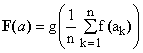The Hölder means, for example, follow from (1) by setting f(x) = xp. Other important special case are:

Exponential mean, defined on the whole of R, obtained by setting f(x) = exp(x), g(x) = ln(x).
Inverse exponential mean, also defined on R, obtained by setting f(x) = exp(-x), g(x) = -ln(x).
Logarithmic mean, defined on R+, obtained by setting f(x) = ln(x), g(x) = exp(x).

However, the logarithmic mean is identical to the geometric mean (equation 2) and thus does not represent anything new, while the exponential and inverse exponential means do not satisfy condition (m2) and thus are likely to be of less use in practical applications, unless the averaged quantities are dimensionless. They are examples of the imperfect means defined above.

However, there is a simple way of removing the imperfection. When M(a) is any selected mean, then the following formula defines a new, normalized generalized mean which satisfies all three conditions:

(18)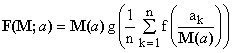Further generalization is possible using two means (not necessarily different ones) M(a) and M'(a), and setting

(19)   F(a) = M(a) g(M'({f(ak/M(a)})).

Expression (18) is in fact a special case of (19) with M'(a) being the arithmetic mean.

Using equations (6), (8) and (19) in various combinations and in an iterative way, one can define an absolutely bewildering number of distinct composite and generalized means starting from a few pre-defined ones.

***** Application-defined means

In practice, means arise in various contexts such as statistics, statistical physics, geometry, numeric approximations of functions, and many more. To illustrate the process, let us see just one example taken from geometry.

Consider an n-dimensional ellipsoid with semi-axes {ak}, k=1,2,...,n. In many applications one is interested in either the "volume" or the "surface" of such an ellipsoid. The numeric expressions for these quantities may be more or less difficult to derive. In this example, the volume presents no problem, while the surface involves transcendental functions and presents considerable computational problems even for n = 2. In any case, however, one can introduce the concept of an "equivalent sphere", i.e., an n-dimensional sphere which has either the same volume or the same surface.

It can be easily shown that the radii of such equivalent spheres are means of the semi-axes {ak}. Naturally, volume and surface lead to different kinds of means. The equivalent volume radius turns out to be the geometric one G(a), while for surface there does not exist any generic formula. However, the definition holds and is perfectly valid; we can denote the radius of the sphere with equivalent surface E({ak}) and call E(a) the elliptic mean of a.

Whether we know how to compute the elliptic mean has nothing to do with its legitimacy; our inability to do so just indicates that it is probably neither efficient nor thrifty (and not a wrapper). What one is strongly tempted to do in these cases is to approximate the new mean arising from an "application" by means of other means which are more conventional and easier to compute. The example of ellipse perimeter (n=2) shows that even that can be a complex task.

APPENDIX: Proofs

A generic Note regarding the proofs of monotonicity, continuity and regularity:
In virtue of (m3), for a set a containing a single element a (n=1), any mean M is equal to a and is therefore monotonous, continuous and regular. It follows that the validity of these properties for any a can be proved by showing that, assuming they hold for all n-tuplets a, they hold also for a generic (n+1)-tuplet {a,x}. Assuming that the symmetry under permutations (m1) has been already proved, it suffices to discuss them only with respect to the added element x.

A generic Note regarding the proofs of coherence and wrapping properties:
It is sufficient to show that, given two sets a, b with na, nb elements, respectively, and their union {a,b}, the following holds:
- Coherence: When na = nb = n, then M({a,b}) = M(M(a),M(b)).
- Wrapping: M is a wrapper if M({a,b}) can be expressed in terms of M(a), M(b) and na, nb.
The generalization to m sets instead of just two by means of a simple recursion is then elementary.

The above Notes and the conventional symbols defined therein are tacitly employed below in the discussions of the respective properties. For example, wherever coherence is discussed, the two sets a and b and their common number of elements n are considered as already defined.

Properties of individual averages and means

***** Minimum & maximum in R
The properties of the algebraic functions min and max of n real variables are assumed to be known.
Proofs are carried out only for min since those for max are analogous:

Properties (m1),(m2),(m3):
m1: Consequence of min(P(a)) = min(a).
m2: Since c is positive, m2 follows from min(ca) = c min(a).
m3: Consequence of min(a) ≤ max(a).

Monotonicity, Continuity and Regularity:
We have min({a,x}) = min(m,x), where m = min(a). Therefore min({a,x}) = x when x ≤ m and min({a,x}) = m for x ≥ m. Consequently, when x is replaced by an x+d, with d > 0, min({a,x}) will increase whenever x < m and remain constant otherwise. Hence min is monotonous, but not strictly monotonous.
Since the derivative D = d[min({a,x})]/dx exists and equals 1 for x < m, and 0 for x > m, min({a,x}) is continuous with respect to x everywhere except perhaps at x = m. But since its right and left limits at x = m exist and are both equal to m, it is continuous everywhere. Being both monotonous and continuous, min is also regular (but not strictly regular).

Simplicity and Stability:
From the above it follows that the equation min({a,x}) = min(a) holds for any x ≥ m.
This implies multiple solutions, so that min is not simple. On the other hand, since x = min(a) is a solution, it is stable.

Coherence:
Since min({a, b}) = min({min(a),min(b)}), min is coherent.

Wrapping:
The latter identity is independent of the number of elements in a, b and thus can be used as the wrapping expression.
Consequently, min is a wrapper.
----------------------------------

***** Median in R:

Properties (m1),(m2),(m3):
m1: Consequence of the fact that the a-priori sorting removes any possible permutation dependence.
m2: Consequence of the defining formulae which are linear in terms of the elements of a.
m3: Consequence of the fact that, for any index k, min(a) ≤ ak ≤ max(a).

Monotonicity, Continuity and Regularity:
1) Let n be odd. Denote a' = a(n-1)/2, a* = a(n+1)/2, and a'' = a(n+3)/2 so that, by definition, a' ≤ a* ≤ a'' and med(a) = a*.
Now add an element x to form a new set {a,x} with an even number of elements and determine its median
(see the Figure on the right, where the new median is marked by a dot. The following situations can occur: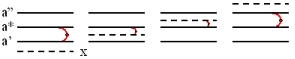a) When x ≤ a', med({a,x}) = (a'+a*)/2
b) When a' ≤ x ≤ a*, med({a,x}) = (x+a*)/2
c) When a* ≤ x ≤ a'', med({a,x}) = (x+a*)/2
d) When x ≥ a'', med({a,x}) = (a''+a*)/2
Consequently, the derivative D = d[med({a,x})]/dx exists everywhere except at the points a' and a''.
In particular, D = 0 when x < a' or x > a'', and D = 0.5 when a' < x < a''.
The mapping, however, is continuous in x since at the special points a' and a'' its left and right limits exist and are identical.
2) Now let n be even and write a' = an/2 and a'' = a1+n/2 so that, by definition, a' ≤ a'' and med(a) = (a'+a'')/2.
Add an element x to form a new set {a,x} with an odd number of (n+1) elements and determine its mean
(see the illustration on the right). In this case, three situations can occur: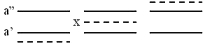a) When x ≤ a', med({a,x}) = a'
b) When a' ≤ x ≤ a'', med({a,x}) = x
c) When x ≥ a'', med({a,x}) = a''
Consequently, the derivative D exists everywhere except at the points a' and a''.
In particular, D = 0 when x < a' or x > a'', and D = 1 when a' < x < a''.
The mapping, however, is continuous since at the special points a' and a'' its left and right limits exist and are identical.
Combining 1) and 2), we see that med is always continuous and monotonous (since D is never negative), though not strictly monotonous (there are cases when D is null). Consequently, it is regular, but not strictly regular.

Simplicity and Stability:
From the above it follows that when x = med(a) then, for both odd and even n, the applicable case is (b) and med({a,x}) = med(a). On the other hand, there are special cases when the equation med({a,x}) = med(a) admits multiple solutions (consider, for example, even n, a' = a'', and any x < a'). Consequently, the median is stable, but not simple.

Coherence:
The following counter-example proves that median is not a coherent mean: Let a = {1,3} and b = {2,6}.
Then med({a,b}) = med({1,2,3,6}) = 2.5 differs from med({med(a),med(b)}) = med({2,4}) = 3.

Wrapping:
There does not exist any generally valid wrapping expression for the median. At best it is possible to prove that when med(a) ≤ med(b), then med(a) ≤ med({a, b}) ≤ med(b).
In particular, if the medians of two sets are the equal, the median of the combined set has also the same value.
----------------------------------

***** Arithmetic mean in R:

Properties (m1),(m2),(m3):
m1: Consequence of the fact the sum of n elements does not depend upon their order (commutativity of addition in R).
m2: Consequence of the identity Σkc ak = c Σkak.
m3: Consequence of the fact that n min(a) =  Σkmin(a) ≤ Σkak ≤ Σkmax(a) = n max(a).

Monotonicity, Continuity and Regularity:
The derivative D = d[A({a,x})]/dx = 1/(n+1) exists everywhere and is positive.
Consequently, the arithmetic mean is strictly monotonous and continuous, and therefore also strictly regular.

Simplicity and Stability:
For any a, the equation A({a,x}) = A(a) has a unique solution x = A(a). To prove this, use directly Eq.(1).
Arithmetic mean is therefore simple and stable.

Coherence:
From Eq.(1) we have A({a,b}) = (Σak+Σbk)/2n = ((Σak)/n+(Σbk)/n)/2 = A({A(a),A(b)}).
Arithmetic average is therefore coherent.

Wrapping:
Again from Eq.(1) we have A({a,b}) = (Σak+Σbk)/(na+nb) = (naA(a)+nbA(b))/(na+nb).
Arithmetic average is therefore a wrapper.
----------------------------------

***** Robust mean R(f,a) in R:

In a robust mean a certain number of largest and smallest elements gets eliminated from the set a and then the arithmetic mean is computed for the remaining subset a'.

Properties (m1),(m2),(m3):
m1: Consequence of the fact that the a-priori sorting removes any possible permutation dependence.
m2: A linear scaling does not change the order of elements and therefore does not affect the elements which are eliminated, leaving invariant also the subset a'. For the rest, refer to the arithmetic mean.
m3: Refer again to the arithmetic mean and consider that, since a' is a subset of a, min(a) ≤ min(a') and max(a) > max(a').

Monotonicity, Continuity and Regularity: We may assume without loss of generality that a is sorted: a1 ≤ a2 ≤ ... ≤ an. Denote as K the integer part of t(n+1) and n' = n-2K the number of elements of {a,x} remaining after the elimination of the K largest and K smallest ones. Assume K > 0, because when K = 0, the case reduces to arithmetic mean which is strictly regular.
Three cases can occur:
(1) x < aK. Since x gets eliminated, D = d[R(t,{a,x})]/dx = 0
(2) aK < x < an-K+1: In this case, x is kept, R(t,{a,x}) = A({aK+1,...,an-K},x) and D = 1/n' (see the arithmetic mean)
(3) x > an-K+1: Since x gets again eliminated, D = 0;
Continuity at x = aK follows from the fact that at that particular point it does not matter whether one eliminates x or aK. The same applies also to the second special point x = an-K+1, thus assuring continuity everywhere. Robust means are therefore regular but, due to the existence of intervals with D = 0, not strictly regular.

Simplicity: Suppose that K>1 and all the elements aK through an-K+1 are equal to a common value a (while this is not true for ak with k<K or k>(n-K+1). Then R(t,{a,x}) = R(t,a) for any x (alternatively, use a counter-example with a = {1,2,2,2,3} and t=0.3).
Equation (9) therefore does not always have a unique solution and, consequently, robust statistics are not simple.

Stability: When the number of eliminated elements in the set a differs from the one in {a,x}, i.e., when [fn] < [t(n+1)] then, barring a fortuitous coincidence, the value x = R(a) is not a solution R({a,x}) = R(a). Consequently, robust means are not stable.
Example: When t = 0.25 and a = {1,2,6}, R(a) = 3, but R({a,3}) = R({1,2,6,3}) = 2.5.

Coherence: If one does not know all the elements of the partial sets, there is no way to sort the combined set e' and determine which of its elements are to be eliminated. Consequently, robust means are not coherent.

Wrapping: No generally valid wrapping formula can be devised. Consequently, robust means are not wrappers.
----------------------------------

***** Geometric mean in R+:

In a certain sense, the geometric mean is a special case of the Hölder mean and therefore the proofs could be referred to the latter. However, the reduction from Hölder mean to geometric involves a limit which might complicate the proofs and raise doubts about their legitimacy. For this reason, independent and explicit proofs are presented.

Properties (m1),(m2),(m3):
m1: Consequence of the fact the product of n elements does not depend upon their order (commutability of multiplication in R).
m2: Use direct substitution of all ak by fak in Eq.(2).
m3: Consequence of the elementary fact that min(a)^n ≤ Πk=1,nak ≤ max(a)^n.

Monotonicity, Continuity and Regularity:
The derivative D = d[G({a,x})]/dx = [G({a,x})/(n+1)]/x exists and is positive everywhere in R+.
Consequently, the geometric mean is therein strictly monotonous and continuous, and therefore also strictly regular.

Simplicity and Stability:
For any set a in R+, the equation G({a,x}) = G(a) has a unique solution x = G(a). To prove this, use directly Eq.(2).
Geometric mean is therefore both simple and stable.

Coherence:
From Eq.(2) we have G({a,b}) = [(Πak).(Πbk)]^[1/2n] = [(Πak)^(1/n).(Πbk)^(1/n)]^[1/2] = G({G(a),G(b)}).
Geometric average is therefore coherent.

Wrapping:
Again from Eq.(2) we have G({a,b}) = [(Πak).(Πbk)]^[1/(na+nb)] = [(G(a))^na.(G(b))^nb]^[1/(na+nb)].
Geometric average is therefore a wrapper.

Geometric mean in R0+:

When 0 is admitted as an element of the domain, geometric mean is no longer simple; whenever any of the elements of a is zero then G(a) = 0 and the equation G({a,x}) = G(a) =0 holds for any x. Moreover, the mean is no longer strictly regular (though it is still regular). Somewhat suprisingly, however, other properties are maintained.
----------------------------------

Quadratic mean is the same as the Hölder mean H(2,a) and shares its basic properties.
----------------------------------

***** Harmonic mean in R+

Harmonic mean is the same as the Hölder mean H(-1,a) and shares its basic properties.
----------------------------------

***** Hölder means in R+

For simplicity, we first assume p ≠ 0. The case p = 0 defaults to the already handled case of geometric mean. The limit transition to p→0 is discussed below, but not used for the analysis of the geometric mean properties (though it would be possible).

Properties (m1),(m2),(m3):
m1: Consequence of the commutability of addition in R+).
m2: Use direct substitution of all ak by fak in Eq.(4).
m3:
a) For positive  p, [min(a)]^p ≤ [ak]^p for any k; hence [min(a)]^p ≤ [H(p,a)]^p and, consequently, min(a) ≤ H(p,a).
b) For negative p, [min(a)]^p ≥ [ak]^p for any k; hence [min(a)]^p ≥ [H(p,a)]^p and, again, min(a) ≤ H(p,a).
Analogous procedures apply to max(a).

Monotonicity, Continuity and Regularity:
The derivative D = d[H(p,{a,x})]/dx = [x/H(p,{a,x})]^(p-1)/(n+1) exists everywhere and is positive in R+.
Consequently, Hölder means are strictly monotonous and continuous, and therefore also strictly regular.

Simplicity and Stability:
For any a, the equation H(p,{a,x}) = H(p,a) has a unique solution x = H(p,a). To prove this, use directly Eq.(4).
Hölder means are therefore simple and stable.

Coherence:
From Eq.(4) we have H(p,{a,b}) = H(p,{H(p,a),H(p,b)}).
Hölder means are therefore coherent.

Wrapping:
Again from Eq.(4) we have H(p,{a,b}) = [[na(H(p,a))^p+nb(H(p,b))^p]/(na+nb)]^(1/p).
Hölder means are therefore wrappers.

Geometric mean as a limit of the Hölder mean H(p,a) for p→0
Write the logarithm of H(p,a) as F(p)/p, where F(p) = ln[Σk=1,n (ak^p)] - ln(n) is a continuous function of p and F(0) = 0. For p→0, the ratio becomes of the type 0/0 but, since the derivatives of both its nominator and its denominator exist, it can be evaluated using the L'Hopital rule. In particular, dF(p)/dp = [Σ(ak^p) ln(ak)]/[Σ(ak^p)] which for p→0 has a well-behaved limit of [Σln(ak)]/n. Hence limp→0 ln(H(p,a)) = [Σln(ak)]/n and therefore H(0,a) = (Πak)^(1/n) = G(a), QED.

Hölder mean inequalities: Proof of inequality (12)

The following proof is a simplified version of the one in Wikipedia, based on Jensen's inequality for convex functions:
Assume first that 0 < p < q. Then f(x) = x^(q/p) is a power of x with exponent greater than 1 and therefore a convex function. One can therefore apply the Jensen's inequality f(Σyk) ≤ Σ[f(yk)] and set yk = ak^p. The result is [Σak^p]^(q/p) ≤ Σak^q or, equivalently, [Σak^p]^(1/p) ≤ [Σak^q]^(1/q). Suppose now that p < q < 0. We set g(x) = x^(p/q) which is again a power of x with exponent greater than 1 and therefore convex. Setting zk = ak^q in the Jensen's inequality g(Σzk) ≤ Σ[g(zk)], one now obtains [Σak^q]^(p/q) ≤ Σak^p or, since p is negative, [Σak^q]^(1/q) ≥ [Σak^p]^(1/p). This proves that H(p,{a}) is a non-decreasing function for p > 0 as well as for p < 0. But the existence of the limit for p→0 (see the preceding para) implies that H(p,{a}) is continuous at p = 0 and therefore non-decreasing everywhere, QED.

Proof of inequality (13) exploits the just proven fact that dH(p,a)/dp ≥ 0. The derivative is quite easy to evaluate explicitly, obtaining dH(p,a)/dp = [H(p,a)/((p^2)(Σak^p))][Σ(ak^p)ln(ak^p)-(Σak^p)ln(Σ(ak^p)/n)]. Since the first term in square brackets is evidently positive, the condition implies that the second term must be non-negative. But the positive numbers ak are arbitrary; consequently, ak^p can be replaced, without any loss of generality, by zk. Hence inequality (13).

Hölder means in R0+:

When 0 is admitted as an element of the domain and p is not positive, Hölder means are no longer simple; whenever any of the elements of a is zero then H(p,a) = 0 and the equation H(p,{a,x}) = H(p,a) =0 holds for any x. Moreover, the mean is no longer strictly regular (though it is still regular). Like in the case of geometric mean, however, other properties are maintained.
----------------------------------

***** Lehmer means in R+:

Properties (m1),(m2),(m3):
m1: Consequence of the commutability of addition in R.
m2: Algebraic consequence of the defining Equation (5).
m3: Algebraic consequence of the fact that in R+, min(a) Σak^(p-1) ≤ Σak^p ≤ max(a) Σak^(p-1).

Monotonicity, Continuity and Regularity:
The derivative D = d[L(p,{a,x})]/dx is easy to compute and exists everywhere. Consequently, Lehmer means are continuous. However, the derivative is not always positive. In fact, here is a counter-example to Lehmer means monotonicity: when e = {0.25,1} and e' = {0.5,1}, then L(2,e) > L(2,e'). In general, therefore, Lehmer means are neither monotonous, nor regular.

Simplicity and Stability:
For any a, the equation L(p,{a,x}) = L(p,a) has a unique solution x = L(p,a). To prove this, use directly Eq.(5).
Lehmer means are therefore simple and stable.

Coherence:
From Eq.(5) it is evident that, except for a fortuitous coincidence, L(p,{a,b}) does not equal L(p,{L(p,a),L(p,b)}).
Lehmer averages are therefore not coherent.

Wrapping:
No generally valid wrapping formula can be devised. Consequently, Lehmer means are not wrappers.

Lehmer mean inequalities: Proof of inequality (15)

It is sufficient to show that for any set of n positive numbers a, the derivative dL(p,a)/dp is non-negative.  Explicit evaluation gives dL(p,a)/dp = [ΣkΣl(ak^p)(al^(p-1))[ln(ak)-ln(al)]] / [Σ(ak)^(p-1)]^2. Since the denominator is a square, the sign of the expression is determined by its nominator, which can be rewritten as ΣΣk<l[(akal)^(p-1)][ak ln(ak/al) + al ln(al/ak)]]. But, given any two positive numbers x,y, the expression x.ln(x/y)+y.ln(y/x) is always non-negative (hint: write it as y.(z-1).ln(z), where z=x/y). Hence all the terms in the nominator of dL(p,a)/dp are non-negative and so is the derivative itself.

Inequalities (16) follow from the identity L(p,a) = H(p,a)^p / H(p-1,a)^(p-1) which implies L(p,a) / H(p,a) = r^(p-1), where r = H(p,a) / H(p-1,a) ≥ 1 due to the Hölder mean inequality (12).

Lehmer means in R0+:

When 0 is admitted as an element of the domain and p is not positive, Lehmer means are no longer simple; whenever any of the elements of a is zero then L(p,a) = 0 and the equation L(p,{a,x}) = L(p,a) =0 holds for any x. However, even in this case, it is still stable.

Compositions of averages and means

***** Composition theorem:

Condition M1: M(Pe) = M0({Mi(Pe)}) = M0({Mi(e)}) = M(e).
Condition M2: M(ce) = M0({Mi(ce)}) = M0({c Mi(e)}) = M0(c{Mi(e)}) = c M0({Mi(e)}) = c M(e).
Condition M3:
a) M(e) = M0({Mi(e)}) ≥ min({Mi(e)}) ≥ min({min(Mi(e))}) ≥ min(e).
b) M(e) = M0({Mi(e)}) ≤ max({Mi(e)}) ≤ max({max(Mi(e))}) ≤ max(e).

Condition M1: M(Pe) = M0({wiMi(Pe)}) = M0({wiMi(e)}) = M(e).
Condition M2: M(ce) = M0({wiMi(ce)}) = M0({wic Mi(e)}) = M0(c{wiMi(e)}) = c M0({wiMi(e)}) = c M(e).

For conditions (M1) and (M2), see the preceding case. Condition M3:
a) M(e) = M0({wiMi(e)}) ≥ M0({wimin(e)}) = min(e) M0({wi}) = min(e).
b) M(e) = M0({wiMi(e)}) ≤ M0({wimax(e)}) = max(e) M0({wi}) = max(e).

***** Generalized means in any suitable definition domain:

First, let us show that expression (17) satisfies conditions (M1) and (M3):
M1) Consequence of the commutability of addition in R.
M3) Since f(x) is a monotonously increasing function, so is its inverse g(x).
We therefore have f(ak) ≥ f(min(a)), [Σ(f(ak)]/n ≥ f(min(a)), and therefore F(a) ≥ g(f(min(a))) = min(a), QED.
Similar reasoning applies to tha max(a) condition.

Now we will prove that when M is a mean, expression (18) is also a mean:
M1) Consequence of the commutability of addition in R and of the fact that M(a) is itself invariant under permutations of a.
M2) Since M(ca) = cM(a), multiplying all ak by a constant c leaves invariant all ak/M(a). Hence F(ca) = cF(a), QED.
M3) Again, since f(x) is monotonously increasing, so is g(x). Now write c = M(a).
We have f(ak/c) ≥ f(min(a)/c), [Σ(f(ak/c)]/n ≥ f(min(a)/c), and therefore F(a) ≥ c.g(f(min(a)/c)) = min(a).
Similar logic applies to tha max condition.

The proof that expression (19) is also a mean follows the same lines as for (18).

 References and Links Means on Math World and Wikipedia. Generalized mean on Wikipedia. This otherwise sound article uses the term "generalized mean" for Hölder mean, a habit I deprecate. Lehmer mean on MathWorld and Wikipedia. Jensen's inequality on MathWorld and Wikipedia. Bullen P.S., Mitrinovic D.S., Vasic M., Means and Their Inequalities, Springer 1999. ISBN 9-027-72629-9. more >> Mathematical Means and Averages: Basic Properties, URL of this article.  Added on 25 July 2009: Sykora S., Mathematical Means and Averages: Generalized Heronian means, Stan's Library, Ed.S.Sykora, Vol.III, 2009.  Added on 16 September 2009: Sykora S., A convex functions inequality, Stan's Library, Ed.S.Sykora, Vol.III, 2009. Discusses a particular set of inequalities on convex and concave functions which apply to Hölder means (it starts with the arithmetic mean, but do not miss the last Section about Extensions).
 TOP | Other math articles in Stan's Library | Math Links Math Books | Stan's HUB |